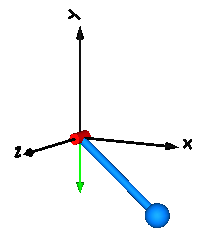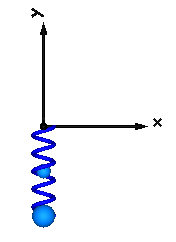# Pendulum–The "Hello World of multi-body dynamics"

This beginners tutorial will model a pendulum pivoted around the origin in the world frame. The world frame is a constant that lives inside the Multibody module, all multibody models are "grounded" in the same world.To start, we load the required packages

using ModelingToolkit
using Multibody, SymbolicIR
using OrdinaryDiffEq # Contains the ODE solver we will use
using Plots

We then access the world frame and time variable from the Multibody module

t = Multibody.t
world = Multibody.world
show(stdout, MIME"text/plain"(), world)
Model world with 12 (18) equations
States (9):
(frame_b₊r_0(t)) [defaults to 0.0]: Position vector directed from the origin of the world frame to the connector frame origin, resolved in world frame
(frame_b₊r_0(t)) [defaults to 0.0]: Position vector directed from the origin of the world frame to the connector frame origin, resolved in world frame
(frame_b₊r_0(t)) [defaults to 0.0]: Position vector directed from the origin of the world frame to the connector frame origin, resolved in world frame
(frame_b₊f(t)) [defaults to 0.0]: Cut force resolved in connector frame
(frame_b₊f(t)) [defaults to 0.0]: Cut force resolved in connector frame
(frame_b₊f(t)) [defaults to 0.0]: Cut force resolved in connector frame
(frame_b₊tau(t)) [defaults to 0.0]: Cut torque resolved in connector frame
(frame_b₊tau(t)) [defaults to 0.0]: Cut torque resolved in connector frame
(frame_b₊tau(t)) [defaults to 0.0]: Cut torque resolved in connector frame
Parameters (4):
n [defaults to 0]: gravity direction of world
n [defaults to -1]: gravity direction of world
n [defaults to 0]: gravity direction of world
g [defaults to 9.81]: gravitational acceleration of world

Unless otherwise specified, the world defaults to have a gravitational field pointing in the negative $y$ direction and an graivational acceleration of $9.81$.

## Modeling the pendulum

Our simple pendulum will initially consist of a Body and a Revolute joint (the pivot joint). We construct these elements by calling their constructors

@named joint = Revolute(n = [0, 0, 1], isroot = true)
@named body = Body(; m = 1, isroot = false, r_cm = [0.5, 0, 0])
┌ Warning: frame_a contains 6 flow variables, yet 3 regular (non-flow, non-stream, non-input, non-output) variables. This could lead to imbalanced model that are difficult to debug. Consider marking some of the regular variables as input/output variables.
└ @ ModelingToolkit /home/github_actions/depots/deepsea1.0/packages/ModelingToolkit/kOxXJ/src/systems/connectors.jl:40
┌ Warning: frame_b contains 6 flow variables, yet 3 regular (non-flow, non-stream, non-input, non-output) variables. This could lead to imbalanced model that are difficult to debug. Consider marking some of the regular variables as input/output variables.
└ @ ModelingToolkit /home/github_actions/depots/deepsea1.0/packages/ModelingToolkit/kOxXJ/src/systems/connectors.jl:40
┌ Warning: frame_a contains 6 flow variables, yet 3 regular (non-flow, non-stream, non-input, non-output) variables. This could lead to imbalanced model that are difficult to debug. Consider marking some of the regular variables as input/output variables.
└ @ ModelingToolkit /home/github_actions/depots/deepsea1.0/packages/ModelingToolkit/kOxXJ/src/systems/connectors.jl:40

The n argument to Revolute denotes the rotational axis of the joint, this vector must have norm(n) == 1. We also indicate that the revolute joint is the root of the kinematic tree, i.e., the potential states of the joint will serve as the state variables for the system.

The Body is constructed by providing its mass, m, and the vector r_cm from its first frame, body.frame_a, to the center of mass. Since the world by default has the gravity field pointing along the negative $y$ axis, we place the center of mass along the $x$-axis to make the pendulum swing back and forth. The body is not selected as the root of the kinematic tree, since we have a joint in this system, but if we had attached the body directly to, e.g., a spring, we could set the body to be the root and avoid having to introduce an "artificial joint".

To connect the components together, we create a vector of connections using the connect function. A joint typically has two frames, frame_a and frame_b. The first frame of the joint is attached to the world frame, and the body is attached to the second joint frame. The order of the connections is not important for ModelingToolkit, but it's good practice to follow some convention, here, we start at the world and progress outwards in the kinematic tree.

connections = [
connect(world.frame_b, joint.frame_a)
connect(joint.frame_b, body.frame_a)
]

With all components and connections defined, we can create an ODESystem like so:

@named model = ODESystem(connections, t, systems=[world, joint, body])

The ODESystem is the fundamental model type in ModelingToolkit used for multibody-type models.

Before we can simulate the system, we must perform model compilation using structural_simplify

ssys = structural_simplify(model, allow_parameter = false)
\begin{align} \frac{\mathrm{d} joint_{+}phi\left( t \right)}{\mathrm{d}t} =& joint_{+}phiˍt\left( t \right) \\ \frac{\mathrm{d} joint_{+}phiˍt\left( t \right)}{\mathrm{d}t} =& joint_{+}phiˍtt\left( t \right) \\ 0 =& joint_{+}n_1 body_{+}frame_{a_{+}tau(t)_1} + joint_{+}n_2 body_{+}frame_{a_{+}tau(t)_2} + joint_{+}n_3 body_{+}frame_{a_{+}tau(t)_3} \end{align}

This results in a simplified model with the minimum required variables and equations to be able to simulate the system efficiently. This step rewrites all connect statements into the appropriate equations, and removes any redundant variables and equations.

We are now ready to create an ODEProblem and simulate it. We use the Rodas4 solver from OrdinaryDiffEq.jl, and pass a dictionary for the initial conditions. We specify only initial condition for some variables, for those variables where no initial condition is specified, the default initial condition defined the model will be used.

D = Differential(t)
defs = Dict(D(joint.phi) => 0, D(D(joint.phi)) => 0)
prob = ODEProblem(ssys, defs, (0, 10))

sol = solve(prob, Rodas4())
plot(sol, idxs = joint.phi, title="Pendulum")

The solution sol can be plotted directly if the Plots package is loaded. The figure indicates that the pendulum swings back and forth without any damping. To add damping as well, we could add a Damper from the ModelingToolkitStandardLibrary.Mechanical.Rotational module to the revolute joint. We do this below

To add damping to the pendulum such that the pendulum will eventually come to rest, we add a Damper to the revolute joint. The damping coefficient is given by d, and the damping force is proportional to the angular velocity of the joint. To add the damper to the revolute joint, we must create the joint with the keyword argument useAxisFlange = true, this adds two internal flanges to the joint to which you can attach components from the ModelingToolkitStandardLibrary.Mechanical.Rotational module. We then connect one of the flanges of the damper to the axis flange of the joint, and the other damper flange to the support flange which is rigidly attached to the world.

@named damper = Rotational.Damper(d = 0.1)
@named joint = Revolute(n = [0, 0, 1], isroot = true, useAxisFlange = true)

connections = [connect(world.frame_b, joint.frame_a)
connect(damper.flange_b, joint.axis)
connect(joint.support, damper.flange_a)
connect(body.frame_a, joint.frame_b)]

@named model = ODESystem(connections, t, systems = [world, joint, body, damper])
ssys = structural_simplify(model, allow_parameter = false)

prob = ODEProblem(ssys, [damper.phi_rel => 1, D(joint.phi) => 0, D(D(joint.phi)) => 0],
(0, 30))

sol = solve(prob, Rodas4())
plot(sol, idxs = joint.phi, title="Damped pendulum")

This time we see that the pendulum loses energy and eventually comes to rest at the stable equilibrium point $\pi / 2$.

## A linear pendulum?

When we think of a pendulum, we typically think of a rotary pendulum that is rotating around a pivot point like in the examples above. A mass suspended in a spring can be though of as a linear pendulum (often referred to as a harmonic oscillator rather than a pendulum), and we show here how we can construct a model of such a device. This time around, we make use of a Prismatic joint rather than a Revolute joint. A prismatic joint has one positional degree of freedom, compared to the single rotational degree of freedom for the revolute joint.@named damper = Translational.Damper(0.5)
@named spring = Translational.Spring(1)
@named joint = Prismatic(n = [0, 1, 0], isroot = true, useAxisFlange = true)

connections = [connect(world.frame_b, joint.frame_a)
connect(damper.flange_b, spring.flange_b, joint.axis)
connect(joint.support, damper.flange_a, spring.flange_a)
connect(body.frame_a, joint.frame_b)]

@named model = ODESystem(connections, t, systems = [world, joint, body, damper, spring])
ssys = structural_simplify(IRSystem(model))

prob = ODEProblem(ssys, [damper.s_rel => 1], (0, 30))

sol = solve(prob, Rodas4())
plot(sol, idxs = joint.s, title="Mass-spring-damper system")

As is hopefully evident from the little code snippet above, this linear pendulum model has a lot in common with the rotary pendulum. In this example, we connected both the spring and a damper to the same axis flange in the joint. This time, the components came from the Translational submodule of ModelingToolkitStandardLibrary rather than the Rotational submodule. Also here do we pass useAxisFlange when we create the joint to make sure that it is equipped with the flanges support and axis needed to connect the translational components.

### Why do we need a joint?

In the example above, we introduced a prismatic joint to model the oscillating motion of the mass-spring system. In reality, we can suspend a mass in a spring without any joint, so why do we need one here? The answer is that we do not, in fact, need the joint, but if we connect the spring directly to the world, we need to make the body (mass) the root object of the kinematic tree instead:

using SymbolicIR
@named root_body = Body(; m = 1, isroot = true, r_cm = [0, 1, 0], phi0 = [0, 1, 0])
@named multibody_spring = Multibody.Spring(c=1)

connections = [connect(world.frame_b, multibody_spring.frame_a)
connect(root_body.frame_a, multibody_spring.frame_b)]

@named model = ODESystem(connections, t, systems = [world, multibody_spring, root_body])
ssys = structural_simplify(IRSystem(model))

defs = Dict(collect(multibody_spring.r_rel_0 .=> [0, 1, 0])...,
collect(root_body.r_0 .=> [0, 0, 0])...,
collect((D.(root_body.phi)) .=> [0, 0, 0])...,
collect(D.(D.(root_body.phi)) .=> [0, 0, 0])...)

prob = ODEProblem(ssys, defs, (0, 30))

sol = solve(prob, Rodas4())
plot(sol, idxs = multibody_spring.r_rel_0, title="Mass-spring system without joint")

Here, we used a Multibody.Spring instead of connecting a Translational.Spring to a joint. The Translational.Spring, alongside other components from ModelingToolkitStandardLibrary.Mechanical, is a 1-dimensional object, whereas multibody components are 3-dimensional objects.

Internally, the Multibody.Spring contains a Translational.Spring, attached between two flanges, so we could actually add a damper to the system as well:

push!(connections, connect(multibody_spring.spring2d.flange_a, damper.flange_a))
push!(connections, connect(multibody_spring.spring2d.flange_b, damper.flange_b))

@named model = ODESystem(connections, t, systems = [world, multibody_spring, root_body, damper])
ssys = structural_simplify(IRSystem(model))
prob = ODEProblem(ssys, defs, (0, 30))

sol = solve(prob, Rodas4())
plot(sol, idxs = multibody_spring.r_rel_0, title="Mass-spring-damper without joint")

The figure above should look identical to the simulation of the mass-spring-damper further above.